# Circles NCERT Solutions : Class 10 Maths

## NCERT Solutions for Class 10 Maths : CirclesFilter
Filters :
Classes
•
•
•
•
•
•
•
Chapters
•
•
•
•
•
•
•
•
•
•
• 4 More
Exercises
•
•
•

### NCERT Class 10 | CIRCLES | Exercise 02 | Question No. 10

Prove that the angle between the two tangents drawn from an external point to a circle is supplementary to the angle subtended by the line-segment joining the points of contact at the centre.### NCERT Class 10 | CIRCLES | Exercise 02 | Question No. 12

A triangle ABC is drawn to circumscribe a circle of radius 4 cm such that the segments BD and DC into which BC is divided by the point of contact D are of lengths 8 cm and 6 cm respectively. Find the sides AB and AC.### NCERT Class 10 | CIRCLES | Exercise 02 | Question No. 09

In Fig. 10.13, X Y and XprimeYprime are two parallel tangents to a circle with centre O and another tangent AB with point of contact C intersecting X Y at A and XprimeYprime at B. Prove that /_A O B"\ "="\ "90oDoubtnut one of the best online education platform provides free NCERT Solutions of Maths for Class 10 Circles which are solved by our maths experts as per the NCERT (CBSE) guidelines. We provide the solutions as video solutions in which concepts are also explained along with answer which will help students while learning , preparing for exams and in doing homework. These Solutions will help to revise complete syllabus and score more marks in examinations.

Get here free, accurate and comprehensive NCERT Solutions for Class 10 Maths Circles which have been reviewed by our maths counsellors as per the latest edition following up the CBSE guidelines. We provide video solutions in which solutions to all the questions of NCERT Class 10 Maths textbook are explained in step by step and detailed way.

## The Topics of the Chapter Circles are :

LENGTH OF THE TANGENT

QUESTIONS

SOME PROPERTIES OF TANGENT TO A CIRCLE

CONSTRUCTION OF TANGENTS TO A CIRCLE

It contains these exercises along with solved examples. All the exercises are solved in the video. Select the exercise to view the solutions exercisewise of the Chapter Circles:

## NCERT Solutions Class 10 Maths Chapter Circles Exercises:

We have covered all the exercises and also Solved examples in the videos. Along with the practise exercise Students should also practise solved examples to clear the concepts of the Circles. If incase you have any doubt you can watch the solutions for the given questions.Watch the solutions for the given questions in which it is also explained in the video steps to solve the questions along with answers.

In the previous chapter, we have learned about some applications of trigonometry. Here, we will learn about one of the most important geometrical shape that is Circle.  A circle is a round shape having a center point equidistant from all the points forming circle. The shape of circle is important because we use them in our everyday lives. You observe them in trucks, cars, bicycles and even our clocks. You cannot escape them! Keeping the significance of circles in mind, in this chapter we will learn topics such as tangent to a circle and number of tangents from a point on a circle. Students can learn how to work on different solutions on Circles in the most appropriate way. Doubtnut has provided the best online study materials where it becomes possible to get the right idea on the different topics swiftly.

CBSE curriculum utilizes NCERT books for imparting quality education to the students. However, NCERT math textbook is useful only in clearing the basic concepts as it lacks ample solved examples. Students do not get the in-depth knowledge of the different topic for which it becomes difficult to attempt the tricky questions which are set in the examination. So, they make their effort to find different guide books in order to clear their concepts. Moreover, in Maths, students should be able to clear all their queries without which it becomes difficult to score good marks. By practicing different exercises, it can help the students to get the right idea about it and achieve success in the perfect manner.

The expert team of Doubtnut has made it possible for the students to get the right and clear idea about the different topics and that too in the easiest manner. They have come up with the most useful online video tutorials that help the students in finding the right idea on different topics. It has also provided Class 10 Maths NCERT Solutions that proves to be an added advantage to them. This helps them to get the best idea on how to attempt the different questions in the easiest manner without any worry at all. With step by steps instructions provided by the experts, it truly proves to be of much use to the students in order to get the right idea on how to attempt the different questions in the easiest manner. Moreover, the NCERT maths solutions are also presented in PDF format. Students can download pdf files to access NCERT solutions anytime and anywhere.

## Topics and subtopics included in NCERT Solutions for Class 10 Maths Chapter 10 Circles

### Introduction

You have learned about circles and other various terms such as segment, chord, sector, etc.  Now let us consider a circle and a line PQ.  In the below figure, there can be three possibilities: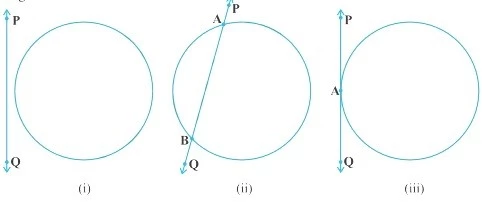In Figure (i), there is no common point between the line PQ and the circle. So, PQ is called a non-intersecting line with respect to the circle.

In Figure (ii), A and B are two common points which the line PQ and the circle have.

In Figure (iii), A is the only point which is common to the line PQ and the circle. So, this is known as a tangent to the circle.

### Tangent to a Circle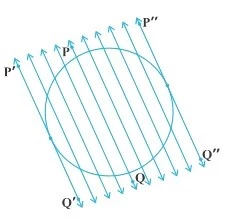In order to get the right knowledge on the existence of the tangent to a circle at a point, let’s have a look at the activity below:

Take a paper and draw a circle along with secant PQ of the circle. Next, try to draw various lines parallel to the secant where it should be on the both sides of it. You will find that after some steps, the length of the chord cut by the lines will gradually decrease, i.e., the two points of intersection of the line and the circle are coming closer and closer.

In one case, you can find that it has become zero on one side of the secant while in another case, you can find that it has becomes zero on the other side of the secant. See the positions P′Q′ and P′′Q′′ of the secant in the figure. These are tangents.

Thus, a tangent is a secant when both of the end points of the corresponding chord coincide. When it comes to the term, point of contact’, it is the common point of the tangent and the circle.

### Another activity is needed so as to get an idea of the number of tangents from a point on a circle.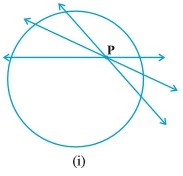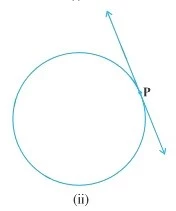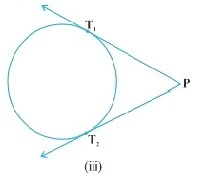Draw a circle on a paper. Take a point P inside it. Is it possible to draw a tangent to the circle through this point? It can be seen that the point intersect the circle in two points. So, it is not possible to draw any tangent to a circle through a point inside it [see Figure (i)].

Next, take a point P on the circle and draw tangents through this point. You have already observed that there is only one tangent to the circle at such a point [see Figure (ii)].

So, now you need to take a point P outside the circle and then make your best effort in trying to draw tangents to the circle from this point. What do you observe? You will find that you can draw exactly two tangents to the circle through this point [see Figure (iii)].

We can summarise these facts as follows:

Case 1: There is no visible tangent to a circle that is seen passing through a point lying inside the circle.

Case 2: There is presence of one and only one tangent to a circle that is seen passing through a point lying on the circle.

Case 3: There are exactly two tangents to a circle through a point lying outside the circle. In Fig. 10.6 (iii), T1and T2 are the points of contact of the tangents PT1 and PT2 respectively.

The segment length from the external point P to the tangent and the point of contact with the circle is called the length of the tangent from the point P to the circle.

Note that in Figure (iii), PT1 and PT2 are the lengths of the tangents from P to the circle. When it comes to PT1 and PT2, they have a common property.

## Role of Doubtnut for Class 10 students

Subject matter experts of Doubtnut have really made it possible to provide the right idea to the students as to how to come up with the right solutions for even complex problems.  There are lot of advantages that students can enjoy once they look forward to the best and useful study materials online. The NCERT Solutions for Class 10 Maths Chapter 10 Circles provided by them helps a lot to get the perfect and clear idea on how to tackle the different questions. Let’s have a look at some of the advantages:

• Lots of examples and exercises included: In order to make the students get their queries cleared, the experts have included lots of exercises as well as examples. This makes it much easier for them to understand the different topics in the simplest manner. So, with the best attempt made by them, it becomes possible to get the perfect idea on how to work on the different problems without any difficulties.
• Learn at your own pace: Students can download the study materials in the form of pdf. This helps them to study at their own pace no matter whether they are at home or even traveling. Therefore, the right decision made by the students to opt for the video tutorials by Doubtnut can really help a lot to achieve success in the exams.
• Get the best support: Finding the best support is also possible from Doubtnut where the students can always expect to get a clear idea about the different topics. This leads to scoring good marks in the examinations where the students would never have to stay ignorant at all. So, by making the best use of the video tutorials, it can help a lot to provide the best education to achieve success in the perfect manner.
• Zero cost solutions: With the aim of helping students from different economic and social backgrounds to have equal opportunity in attaining the quality education, Doubtnut has provided it solutions at zero cost, the students do not have pay any money to access the study material on our website and playstore app.

Share this on :-